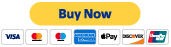###### Deep Learning

Machine Learning Basics

• Linear Algebra, Probability Distributions, Numerical Computation

• Learning Algorithms, Hyperparameters and Validation Sets

2. Neural Networks

• Introduction to Neural Networks,

• Perceptron Learning, Backpropagation Learning

3. Deep Learning ( Deep Nets )

• Deep Feedforward Networks

• Regularization for Deep Learning

• Convolutional Neural Networks

• Recurrent Neural Networks

4. Applications

• Large-Scale Deep Learning

• Speech Recognition

• Natural Language Processing (NLP)

Tech Stack:

PyTorch ( http://pytorch.org ), TensorFlow ( when needed )Course features:

1. Course instructions

2. Assignments every week

3. Courses are designed to build real-world applications for the MARKETPLACE

Price: \$1499

Duration : 3 months (2 hours a week)